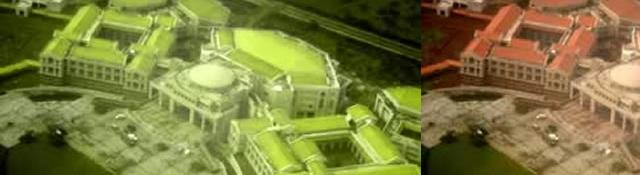## Introduction

In the previous tutorial, Hello World with Images, you learned how to load an image. In this tutorial, we'll take it a step further. You'll do photoshop style "editing" of the image in real time. Things like increasing the brightness, equalizing the histogram, blurring, removing noise, etc. And doing all of this requires only a few lines of code!

## Step 1: Creating the application

Create a new Win32 Console Application, and name it "Filters". Save it wherever you like. Accept the defaults and click Finish.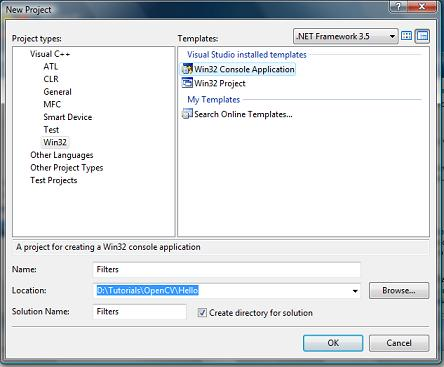This generates a project. Open the file Filters.cpp. We'll modify its code.

At the top, include the CV headers:

```#include <cv.h>
#include <highgui.h>
```

In the main function,

```int main()
{
```

Add these lines to the main function:

```    IplImage* img = cvLoadImage("C:\\\\orangeman.jpg");

cvNamedWindow("Original");

cvShowImage("Original", img);

// We add processing code here

cvWaitKey(0);
cvReleaseImage(&img);
return 0;
}
```

What we're doing here is, loading the file "C:\\orangeman.jpg" into img. Then we create a window, and display the image in it. This is the original image. And then wait till eternity for a key press. As soon as a key is pressed, the image will be released and the program will terminate.

Now add links to the various OpenCV libraries as shown in Step 3 of the Hello World with OpenCV tutorial.

## Step 2: Trying out things

We'll just experiment with the various "tools" available. This will demonstrate the power of OpenCV, and you'll learn about OpenCV as well.

### Part A: Eroding the image

Replace the "// We add processing code" herewith the following code:

```    cvErode(img, img, 0, 2);

cvNamedWindow("Eroded");
cvShowImage("Eroded", img);
```

Notice the cvErode? This function takes in image specified in the first parameter, and outputs an eroded image into the second parameter. The third parameter is mostly for advanced uses, so I won't go into the details about that. The fourth parameter is the number of times you want to erode the image. In my case, I tell it to erode the image twice.

Once we've eroded the image, we create a new window titled "Eroded" and display the image in there. Here's the output I got: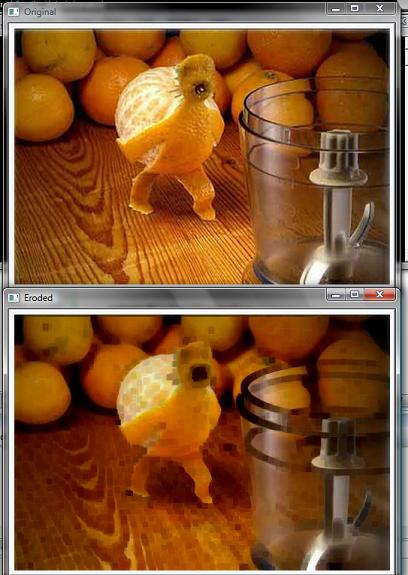Note: cvErode is an in-place function... meaning the input and output images can be same. Not many functions in OpenCV are in-place. Most of them require that the input and output images are different.

### Part B: Dilating the image

Dilating the image is as simple as eroding it. Just replace "Erode" in the above code with a "Dilate"!! Here's the complete code:

```int main()
{
cvNamedWindow("Original");

cvShowImage("Original", img);

cvDilate(img, img, 0, 2);
cvNamedWindow("Dilated");

cvShowImage("Dilated", img);

cvWaitKey(0);
cvReleaseImage(&img);
return 0;
}
```

Again, cvDilate is an in-place function. Here's the result I got on my image:### Part C: Brightness

OpenCV stores images in the form of matrices. Yes, the matrices you studied in high school. If you didn't, a matrix is a grid of values... something like this:In OpenCV's matrix, each pixel can have a value from 0 to 255. So, each pixel has 8-bits of memory alloted to it. If the image is grayscale, it has just one such matrix. So you often hear of 8-bit grayscale images.

If the image is coloured (say a JPG image), it will have 3 matrices. One matrix for the Red component, one for the Green and one for Blue (or, technically speaking, three 8-bit planes). This means each pixel has 3x8 bits alloted to it (hence, you hear of a 24-bit image).

So to increase the brightness of an image, you just add some value to the entire image. If it is a coloured image, you add that value to each of the components.

To add, we use the cvAddS function. This function adds a scalar to each element of the matrix. There also exists a cvAdd function, but that function adds two matrices (not a matrix and a value).

Replace the // We add processing code herewith the following code:

```    cvAddS(img, cvScalar(50,50,50), img);
cvNamedWindow("Bright");
cvShowImage("Bright", img);
```

The cvAddS function takes 3 parameters. The first is the source image. The last is the destination image. The second parameter is the value you want to add to each pixel.

Because this is a coloured image, it will have 3 matrices. And we need to add the constant to each of the 3 planes. So we specify the value to be added for each plane using cvScalar(50, 50, 50).Had this been a grayscale image (with only one matrix), we'd do just a cvScalar(50). However, if you did a cvScalar(50) to a coloured image, you'd get wierd results.... something like this:### Part D: Contrast

For brightness, you added values. For contrast, you multiply.

For adding scalars (values), you use cvAddS. For multiplying, you use cvScale. Here's the code for it... replace the // We add processing code herewith the following code:

```    cvScale(img, img, 2);
cvNamedWindow("Contrast");

cvShowImage("Contrast", img);
```

cvScale's first parameter is the source image, the second is the destination image. And the last parameter is the multiplication value. In this case, each pixel is multiplied by 2. If you multiplied by 1, you won't see any change.

Here's the output I got: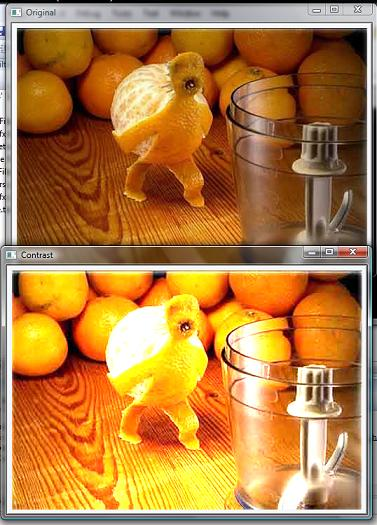### Part E: Negative

Inverting an image is equivalent to doing a logical NOT operation on each element in the matrix. Here's code for doing it... which I'm sure you can work out on your own now:

```    cvNot(img, img);
cvNamedWindow("Invert");
cvShowImage("Invert", img);
```

The cvNot performs a logical NOT on each pixel of the source image (the first parameter) and stores the output in the destination image (the second parameter). Then we create a window, and display the processed image in it: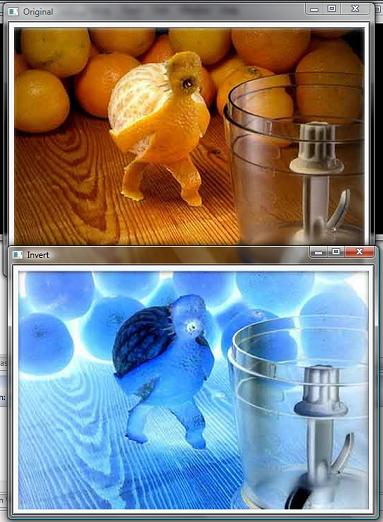## Why not just use Photoshop?

This is a question that is often asked... after all, photoshop has a lot more fancy effects... plastic wrap, lens flare and what not.

With Photoshop, you can modify one image and save it. Or at best, modify a batch of images and save them. But thats it.

With OpenCV, you can take the images in realtime (from a camera) and do things with those images in real time. This is something you simply cannot do with Photoshop.

## Conclusion

I hope you learned quite a bit in this tutorial. In the next tutorial, you'll see how to take input directly from a webcam!

## Next Parts

This post is a part of an article series on OpenCV for Beginners

#### Related posts#### 7 unique neural network architectures#### An introduction to contours#### Greyscale and RGB color spaces

Utkarsh Sinha created AI Shack in 2010 and has since been working on computer vision and related fields. He is currently at Microsoft working on computer vision.